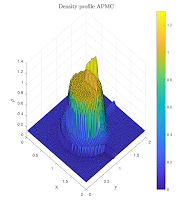### Asymptotic-Preserving Monte Carlo methods for transport equations in the diffusive limitGiacomo Dimarco, Lorenzo Pareschi, Giovanni Samaey (30/7/2017 SIAM J. Sci. Comput. 40 (2018), no. 1, A504–A528. arXiv:1707.09672)

We develop a new Monte Carlo method that solves hyperbolic transport equations with stiff terms, characterized by a (small) scaling parameter. In particular, we focus on systems which lead to a reduced problem of parabolic type in the limit when the scaling parameter tends to zero. Classical Monte Carlo methods suffer of severe time step limitations in these situations, due to the fact that the characteristic speeds go to infinity in the diffusion limit. This makes the problem a real challenge, since the scaling parameter may differ by several orders of magnitude in the domain.
To circumvent these time step limitations, we construct a new, asymptotic-preserving Monte Carlo method that is stable independently of the scaling parameter and degenerates to a standard probabilistic approach for solving the limiting equation in the diffusion limit. The method uses an implicit time discretization to formulate a modified equation in which the characteristic speeds do not grow indefinitely when the scaling factor tends to zero. The resulting modified equation can readily be discretized by a Monte Carlo scheme, in which the particles combine a finite propagation speed with a time-step dependent diffusion term. We show the performance of the method by comparing it with standard (deterministic) approaches in the literature.